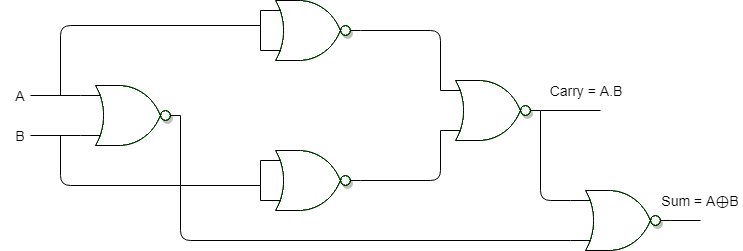# Design a half-adder logic circuit using only nor gate Design a full subtractor logic circuit using only NOR gate

a) Half-adder logic circuit using only nor gate:b) Full subtractor logic circuit using only NOR gate: